# How to Count Cells More Than Certain number of Characters in Excel

This post will guide you how to count the number of cells that more than a certain number of characters in a given range cells using a formula in Excel 2013/2016.How do I count cells with length that greater than a specific number (10) in Excel.

## Count Cells that Contain More Than a Certain Number 10

Assuming that you want to count cells that contain more than a certain number of characters in a selected range(A1:A6).In this case, you can use a formula based on the SUMPRODUCT function and the N function.

Enter the following formula in a blank cell, and press Enter key:

=SUMPRODUCT(N(LEN(A1:A6)>10))

Or

= SUMPRODUCT(–(LEN(A1:A6)>10))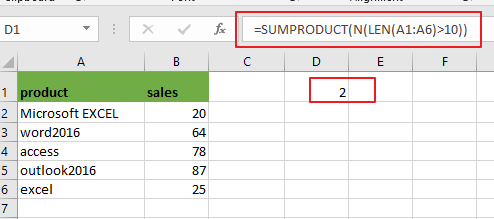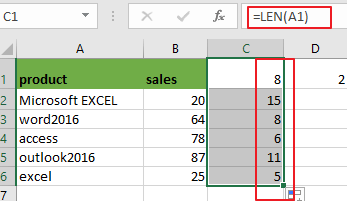Note: A1:A6 is the data range that you want to use. `LEN(A1:A6)>10` is a condition that that data need to be matched.

Now Let’s see how this formula works:

The LEN function will calculate the length for the selected range of cells. Since multiple values were passed into the LEN function and it should be return multiple results as an array list like this: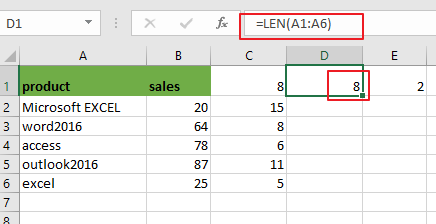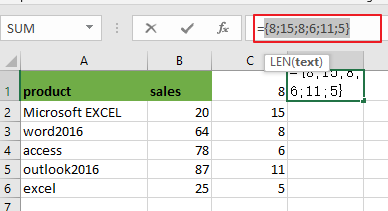={8;15;8;6;11;5}

The LEN function will combine with a logic expression “>10”, and it will return multiple results in an array also like below: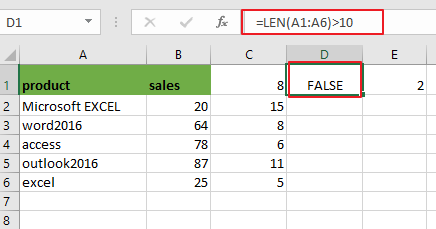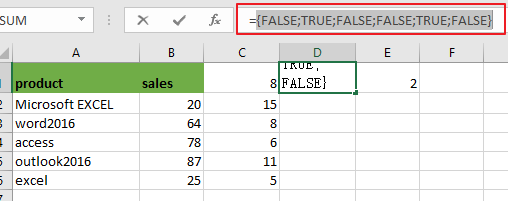={FALSE;TRUE;FALSE;FALSE;TRUE;FALSE}

Then the above array result need to be converted to ones and zeros through the N function or double subtract operators like below:

=–(LEN(A1:A6)>10)

Or

=N(LEN(A1:A6)>10)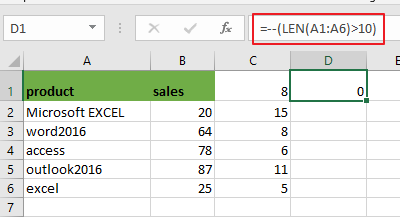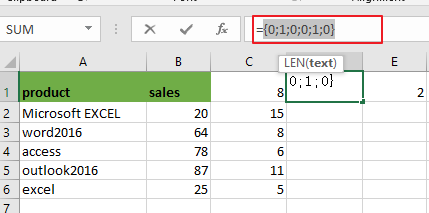Array result is like as below:

={0;1;0;0;1;0}

Last, the above array result contain zeros and ones should be counted by SUMPRODUCT function, it returns 2.

### Related Functions

• Excel SUMPRODUCT function
The Excel SUMPRODUCT function multiplies corresponding components in the given one or more arrays or ranges, and returns the sum of those products.The syntax of the SUMPRODUCT function is as below:= SUMPRODUCT (array1,[array2],…)…
• Excel LEN function
The Excel LEN function returns the length of a text string (the number of characters in a text string).The syntax of the LEN function is as below:= LEN(text)…
• Excel N function
The Excel LEN function returns the length of a text string (the number of characters in a text string).The syntax of the LEN function is as below:= LEN(text)…